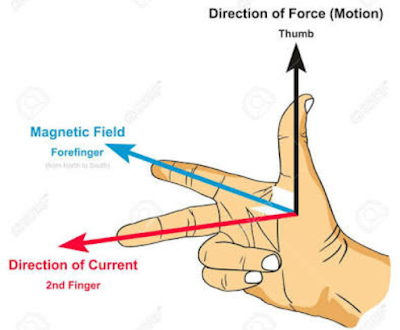# Basic Electrical Theory: The Fundamental Laws of Electricity

0
205

### OHM’S LAW

#### If we plot a graph between Voltage V and current I , then the curve will be a straight line passing through origin. This figure shows the graph between Voltage and Current.Graph of Voltage vs Current.
Slope of the line represents the resistance of the conductor/circuit.

Higher the slope, higher will be the resistance.
Lower the slope, lower will be the resistance.

Note that , Ohm’s law is not an universal law. Materials which obeys Ohm law are called linear materials or ohmic materials.

### LENZ LAW

#### Formula#### For example : As shown in this figure, a bar magnet with the North Pole towards coil is moved towards the coil. A current will be induce in the coil. The direction of current in the coil will be such that it will oppose the motion of the magnet.### FLEMING’S LEFT HAND RULE.

#### If we stretch the fingers of left hand as shown in figure, thenLeft Hand Rule

### FLEMING’S RIGHT HAND RULE.

#### If we strech the fingers of right hand as shown in figure, thenRight Hand Rule